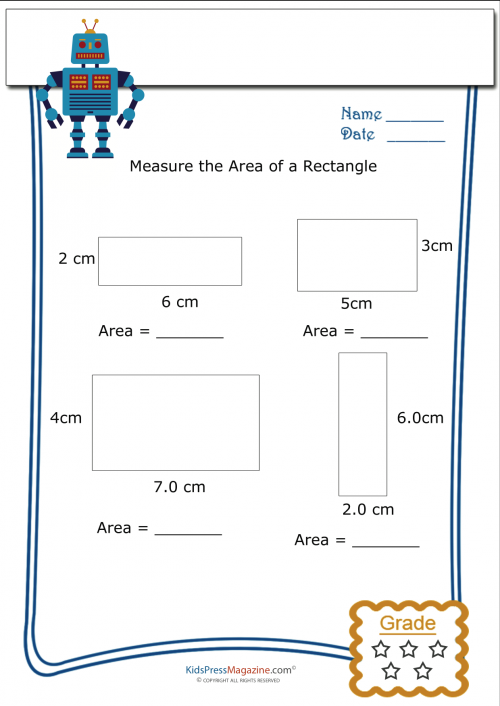•# Measuring Area Worksheet – Rectangle 1

Calculating the area of a rectangle is easy! Teach your child the formula area equals height multiplied by width, or A = H x W

Once your child understands the formula it is all a matter of practice!